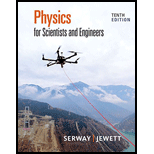Chapter 16, Problem 16PPhysics for Scientists and Enginee...

10th Edition
Raymond A. Serway + 1 other
ISBN: 9781337553278

Solutions

Chapter
SectionPhysics for Scientists and Enginee...

10th Edition
Raymond A. Serway + 1 other
ISBN: 9781337553278
Textbook Problem

In a region far from the epicenter of an earthquake, a seismic wave can be modeled as transporting energy in a single direction without absorption, just as a string wave does. Suppose the seismic wave moves from granite into mudfill with similar density but with a much smaller bulk modulus. Assume the speed of the wave gradually drops by a factor of 25.0, with negligible reflection of the wave. (a) Explain whether the amplitude of the ground shaking will increase or decrease. (b) Does it change by a predictable factor? (This phenomenon led to the collapse of part of the Nimitz Freeway in Oakland, California, during the Loma Prieta earthquake of 1989.)

(a)

To determine

Whether the amplitude of the ground shaking will increase of decrease.

Explanation

The speed of the wave gradually drops by a factor of 25.0.

Formula to calculate the rate of energy transfer is,

P=12μω2A2v        (I)

Here, P is the rate of energy transfer, μ is the linear mass density, ω is the angular frequency, A is the amplitude of the wave and v is the speed of the wave.

The above equation shows that at constant μ and ω,

P=FA2v

Here, F is some constant quantity.

As there is no absorption of energy therefore, the rate of energy transfer is same in granite and mudfill.

Pgran=PmudFAgran2vgran=FAmud2v

(b)

To determine

Whether it change by a predictable factor.

Still sussing out bartleby?

Check out a sample textbook solution.

See a sample solution

The Solution to Your Study Problems

Bartleby provides explanations to thousands of textbook problems written by our experts, many with advanced degrees!

Get Started